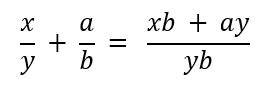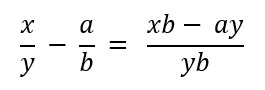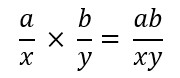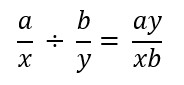# Fraction Calculator

Fraction Calculator allows you to do the addition, subtraction, multiplication, and division of two fraction values. Even more, it supports simple and mixed fractions.

 +  –  ×  ÷

Result:
View:
Calculation:
Related

Fractions are very fascinating and sometimes it is confusing. However, the knowledge of fractions is very important in mathematics. Also, it is helpful for solving real-life math problems.Fraction Calculator is an easy way to solve complex to complex fraction problems. Whether it is addition, subtraction, multiplication, or division.

This calculator allows you to add, subtract, multiply, or divide simple fractions and mixed fractions online. Yes, with this fraction calculating tool you can operate with both mixed and simple fractions.

Read the full post to know how the calculator works. and the procedure to use it. The interface is very simple and user-friendly.

## Why use Fraction Calculator?

There are various reasons why we should not prefer manual calculation over the calculator/tool. Some of the most common and important reasons are:

• It will save you time: Undeniably, if you use a calculator over manual calculation, it consumes less time. You just need to enter the values and press on the “Calculate” button. Subsequently, it’s a very efficient method and long calculations will be completed in seconds.
• Accuracy: When you perform manual calculations, then there are chances that your answers may be incorrect. This is completely the opposite in the case of a fraction calculator. Just because it is pre-programmed, it uses its algorithms to solve each problem without giving any errors.
• Availability: Everywhere you can’t carry a pen or paper to do the manual calculation. In that case, the fraction calculating tool comes into use.

#### How does the Fraction Calculator Work?

So, let’s try to understand the process behind the fraction calculation. Firstly, we will know how fractions are added. Then we will move ahead toward subtraction, multiplication, and division as well. So, read all the mathematical explanations behind it.

The simplest formula used for adding fractions is:Let’s make it more clear through an example. We have to add these two fractions. So, first of all, determine the LCM of the denominators (or multiply them if you don’t get the LCM).

1/3 + 2/5

In this case, we get 15 and place that number in the denominator. After that use the above formula and find the final answer. You can expand this formula to more fractions and add them all together.

(1 x 5) + (2 x 3) / 15 = 11/15

##### Subtraction of Fractions

The formula for subtracting the fractions is similar to the addition method. The difference is just a sign. Everything remains the same as the addition.##### Multiplication of Fractions

Multiplying fractions is very easy to calculate. The formula for multiplying fractions is:Example:

If we multiply 5/2 and 3/8, we will get (5 x 3) / (2 x 8) = 15/16

##### Division of Fractions

For dividing the fractions we implement this formula which is also known as cross multiplication.For Example:

If we divide 5/2 and 3/8, we will get (5 x 8) / (2 x 3) = 40/6 = 20/3

### How to Use?

This is the main section you have been waiting for. Most importantly, you should know that this fraction calculator is an online tool.

In other words, it can only be used when there is an internet connection. If you have an internet connection and a device to access it then you can easily use the tool.

First of all, start your Internet Browser then go to the Fraction Calculator.

Then, select the methods – Simple or Mixed and enter the values in the blank box.

After that, select the operation you want to perform (Addition, Subtraction, Multiplication, or Division) from the list. After that press the “Calculate” button to know the results. The result will appear below along with the calculation.

For reusing the calculator click on the “Reset” button. As a result, all fields will be clear. So, you can recalculate again.